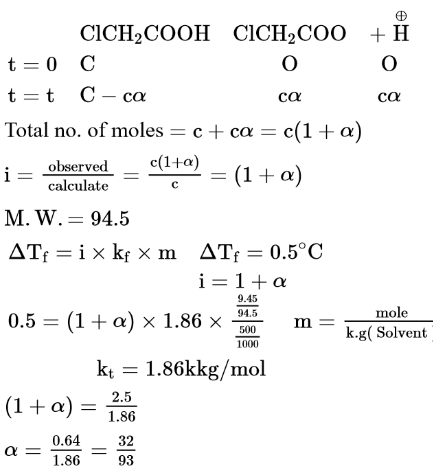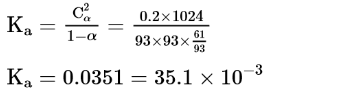# Solve the followingQuestion:

When $9.45 \mathrm{~g}$ of $\mathrm{ClCH}_{2} \mathrm{COOH}$ is added to $500 \mathrm{~mL}$ of water, its freezing point drops by $0.5^{\circ} \mathrm{C}$. The dissociation constant of $\mathrm{ClCH}_{2} \mathrm{COOH}$ is $\mathrm{x} \times 10^{-3}$. The value of $\mathrm{x}$ is _____________(Rounded off to the nearest integer)

$\left[K_{f\left(H_{2} O\right)}=1.86 \mathrm{~K} \mathrm{~kg} \mathrm{~mol}^{-1}\right]$

Solution:

(35)